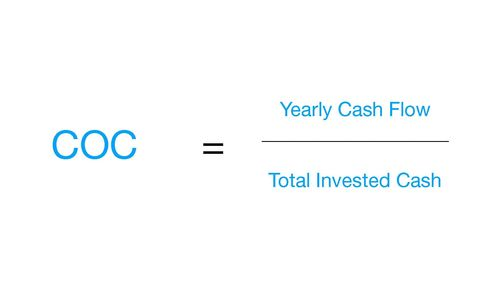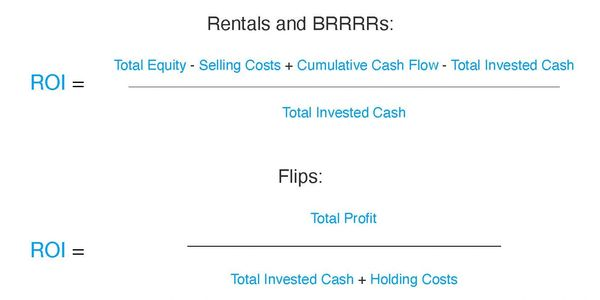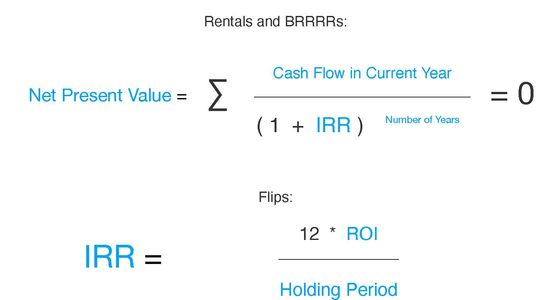A rate of return of a rental property based on comparing the yearly NOI to the purchase price or market value.
Since the cap rate does not take into account loan payments, it can be used to compare rental properties irrespective of financing terms.#### Cash on Cash Return (COC)

A rate of return of a rental property based on comparing the yearly cash flow to the total invested cash.

COC represents the yearly return you will receive on your invested capital.#### Return on Equity (ROE)

A rate of return of a rental property based on comparing the yearly cash flow to your total equity in that property.

ROE represents the yearly return you will receive on your total equity at the end of each year.#### Return on Investment (ROI)

A rate of return of a real estate transaction based on comparing the total profit from your investment to the total invested cash.

For a rental property, the ROI takes into account the cumulative cash flow, equity accumulation and loan paydown and gives the total cumulative return on your invested capital, should you decide to sell the property at a given point in time.

For a flip, the ROI gives the total return on your invested capital after you complete the rehab and sell the property.#### Internal Rate of Return (IRR)

An average annualized rate of return on your total invested cash, sometimes also called annualized ROI.

For a rental property, the IRR takes into account the cumulative cash flow, equity accumulation and loan paydown and gives the annualized return on your invested capital for the entire time you own the property.

Note that the rental IRR formula is not algebraic. Instead, IRR is calculated by solving the formula below when the net present value equals to 0.

For a flip, the IRR is a hypothetical annualized return on your invested capital based on the current flip transaction.